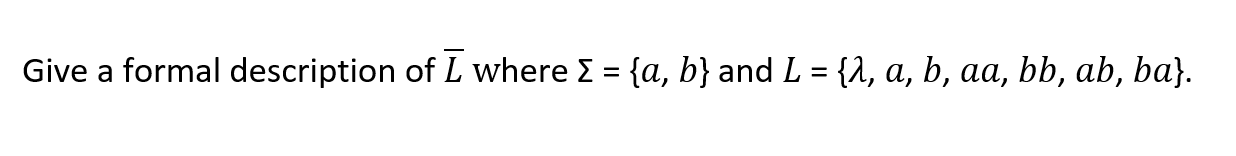Home / Expert Answers / Computer Science / automata-theory-give-a-formal-description-of-bar-l-where-sigma-a-b-and-l-pa263

# (Solved): Automata Theory: Give a formal description of $$\bar{L}$$ where $$\Sigma=\{a, b\}$$ and $$L=\{ ... Automata Theory:Give a formal description of \( \bar{L}$$ where $$\Sigma=\{a, b\}$$ and $$L=\{\lambda, a, b, a a, b b, a b, b a\}$$.

We have an Answer from Expert